# NCERT Solutions for Class 12 Chemistry Chapter 3 Electrochemistry

In this chapter, we provide NCERT Solutions for Class 12 Chemistry Chapter 3 Electrochemistry for English medium students, Which will very helpful for every student in their exams. Students can download the latest NCERT Solutions for Class 12 Chemistry Chapter 3 Electrochemistry pdf, free NCERT solutions for Class 12 Chemistry Chapter 3 Electrochemistry book pdf download. Now you will get step by step solution to each question. Class 12 Chemistry is a very important subject for entrance exams like IIT JEE, CPMT, etc. NCERT Class 12 chemistry notes in Hindi

## NCERT Solutions for Class 12 Chemistry Chapter 3 Electro chemistry

Question 1.
How would you determine the standard electrode potential of the system Mg2+ | Mg?
Solution:
Set up an electrochemical cell consisting of MglMgSO4(1 M) as one electrode by dipping a magnesium wire in 1 M MgSO4 solution and standard hydrogen electrode Pt, H2 (1 atm) | H+(1 M) as the second electrode. Measure the EMF of the cell and also note the direction of deflection in the voltmeter. The direction of deflection shows that the electrons flow from magnesium electrode to hydrogen electrode, i.e., oxidation takes place on magnesium electrode and reduction on hydrogen electrode.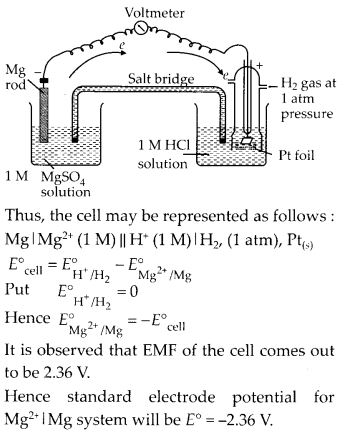Question 2.
Can you store copper sulphate solution in a zinc pot ?

Solution: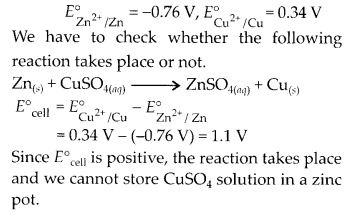Question 3.
Consult the table of standard electrode potentials and suggest three substances that can oxidise ferrous ions under suitable conditions.
Solution:
Oxidation of ferrous ions means :Only those substances can oxidise Fe2+ to Fe3+ which are stronger oxidising agents and have positive reduction potentials greater than 0.77 V so that EMF of the cell reaction is positive. This is for elements lying below Fe3+/Fe2+ in the electrochemical series, for example, Br2, Cl2 and F2.

Question 4.
Calculate the potential of hydrogen electrode in contact with a solution whose pH is 10.

Solution: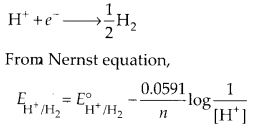Question 5.
Calculate the emf of the cell in which the following reaction takes place :
Ni(S) + 2Ag+ (0.002 M) → Ni2+(0.160 M) + 2Ag(S)
Given that E°cell = 1.05 V

Solution: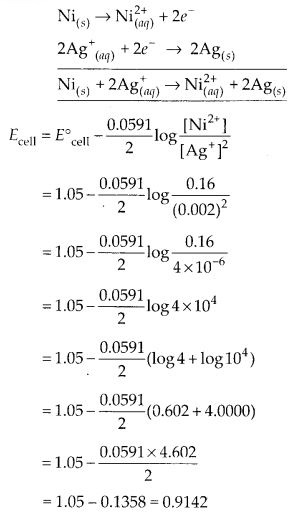Question 6.
The cell in which the following reaction occurs: 2Fe3+(aq) + 2l(aq) → 2Fe2+(aq) + l2(s) has E°cell = 0.236 V at 298 K. Calculate the standard Gibb’s energy and the equilibrium constant of the cell reaction.

Solution: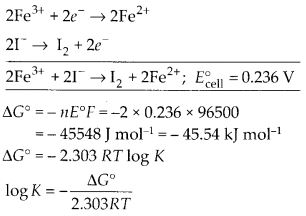Question 7.
Why does the conductivity of a solution decrease with dilution?
Solution:
Conductivity of solution decreases with dilution because number of ions per unit volume decreases.

Question 8.
Suggest a way to determine the A°m value of water.
Solution:
Water is a weak electrolyte. Its A°m value can be determined with the help of Kohlrausch’s law.Question 9.
The molar conductivity of 0.025 mol L-1 methanoic acid is 46.1 S cm2 mol-1. Calculate its degree of dissociation and dissociation constant. Given λ (H+) = 349.6 S cm2 mol-1 and λ (HCOO) = 54.6 S cm2 mol-1

Solution:Question 10.
lf a current of 0.5 ampere flows through a metallic wire for 2 hours, then how many electrons would flow though the wire?

Solution: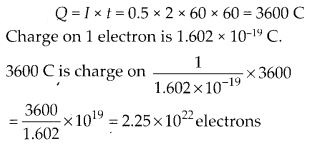Question 11.
Suggest; a list of metals that are extracted electrolytically.
Solution:
Ca, Na, K, Al are extracted electrolytically.

Question 12.
Consider the reaction:
Cr2O2-7 + 14H+ + 6e → 2Cr3+ + 7H2O,
What is the quantity of electricity in coulombs needed to reduce 1 mol of Cr2 O2-7?

Solution: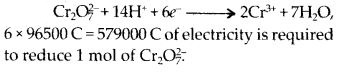Question 13.
Write the chemistry of recharging the lead storage battery, highlighting all the materials that are involved during recharging.
Solution:
Chemical reactions while recharging :
2PbSO4 + 2H2O → PbO2 + Pb + 2H2SO4
Electricity is passed through the electrolyte PbSO4 which is converted into PbO2 and Pb.

Question 14.
Suggest two materials other than hydrogen that can be used as fuels in fuel cells.
Solution:
CH4 and CO can be used in fuel cell instead of hydrogen.

Question 15.
Explain how rusting of iron is envisaged as setting up of an electrochemical cell.
Solution:
The following reactions take place at the surface of iron metal which acts as an electrochemical cell.The water layer present on the surface of iron dissolves acidic oxides of air like C02 to form acids which dissociate to give H+ ions. Fe starts losing electrons in presence of H+ ions.
H2O + CO2 → H2CO3$\rightleftharpoons$ 2H+ + C032-

NCERT Exercises

Question 1.
Arrange the following metals in the order in which they displace each other from the solution of their salts.
Al, Cu, Fe, Mg and Zn.
Solution:
Mg, Al, Zn, Fe, Cu

Question 2.
Given the standard electrode potentials,
K+/ K = – 2.93 V, Ag+/ Ag = 0.80 V, Hg2+/ Hg = 0.79 V, Mg2+/ Mg = -2.37 V, Cr3+/ Cr = – 0.74 V
Arrange these metals in their increasing order of reducing power.
Solution:
Higher the oxidation potential, more easily it is oxidized and hence greater is the reducing power. Thus, increasing order of reducing power will be
Ag < Hg < Cr < Mg < K.

Question 3.
Depict the galvanic cell in which the reaction Zn(S) + 2Ag+(aq) Zn2+(aq), + 2Ag(s) takes place. Further show :

1. Which of the electrode is negatively charged?
2. The carriers of the current i n the cell.
3. Individual reaction at each electrode.

Solution: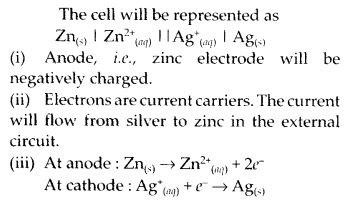Question 4.
Calculate the standard cell potentials of galvanic cell in which the following reactions take place :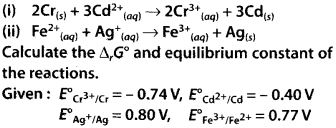Solution: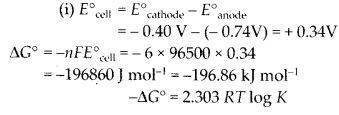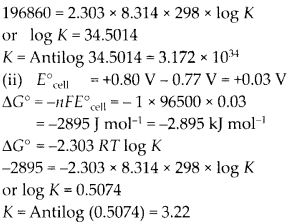Question 5.
Write the Nernst equation and emf of the following cells at 298 K :Solution: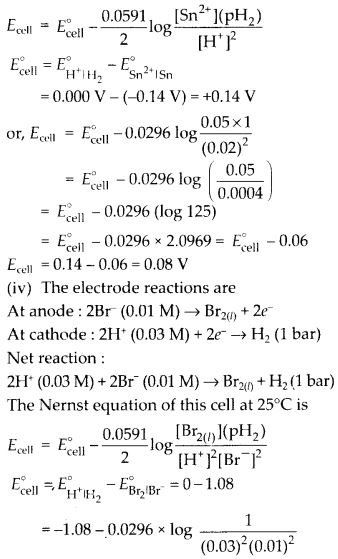Question 6.
In the button cells widely used in watches and other devices the following reaction takes place :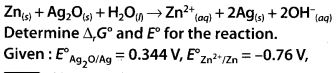Solution:Question 7.
Define conductivity and molar conductivity for the solution of an electrolyte. Discuss their variation with concentration.
Solution:
The reciprocal of resistivity is known as specific conductance or simply conductivity. It is denoted by K (kappa). Thus, if K is the specific conductance and G is the conductance of the solution, then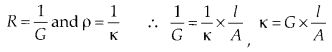Now, if I = 1 cm and A = lsq.cm, then K = G.

Hence, conductivity of a solution is defined as the conductance of a solution of 1 cm length and having 1 sq. cm as the area of cross¬section. Alternatively, it may be defined as conductance of one centimetre cube of the solution of the electrolyte.

Molar conductivity of a solution at a dilution V is the conductance of all the ions produced from 1 mole of the electrolyte dissolved in V cm3 of the solution when the electrodes are one cm apart and the area of the electrodes is so large that the whole of the solution is contained between them. It is represented by ∆m.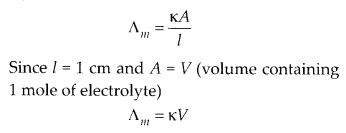Variation of conductivity and molar conductivity with concentration: Conductivity always decreases with decrease in concentration, for both weak and strong electrolytes. This is because the number of ions per unit volume that carry the current in a solution decreases on dilution.

Molar conductivity increases with decrease in concentration. This is because that total volume, V, of solution containing one mole of electrolyte also increases. It has been found that decrease in K on dilution of a solution is more than compensated by increase in its volume.

Question 8.
The conductivity of 0.20 M solution of KCI at 298 K is 0.0248 S cm-1. Calculate its molar conductivity.

Solution:Question 9.
The resistance of a conductivity cell containing 0.001 M KCI solution at 298 K is 1500 Ω. What is the cell constant if conductivity of 0.001 M KCI solution at 298 K is 0.146 × 10-3 S cm-1.
Solution:
Cell constant = K × R = 0.146 × 10-3 × 1500 = 0.219 cm-1

Question 10.
The conductivity of sodium chloride at 298K has been determined at different concentrations and the results are given below:
Concentration/M 0.001 0.010 0.020 0.050 0.100
102 × K/S m-1 1.237 11.85 23.15 55.53 106.74
Calculate ∆m for all concentrations and draw a plot between ∆m and c1/2. Find the value of ∆°m

Solution: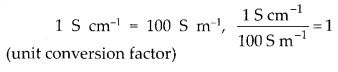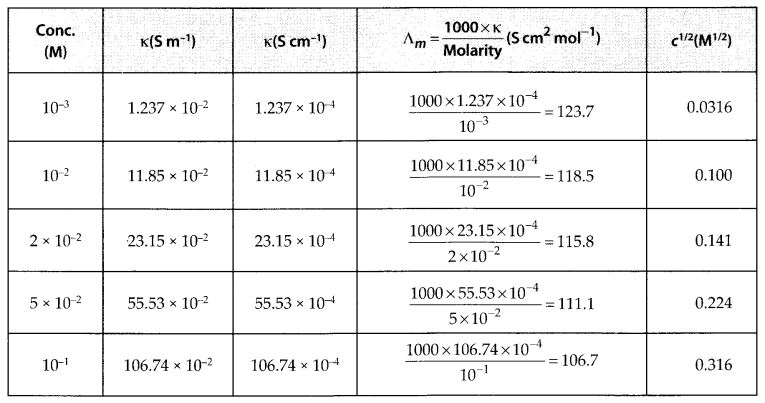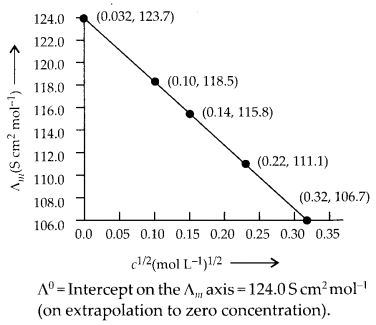Question 11.
Conductivity of 0.00241 M acetic acid is 7.896 × 10-5 S cm-1. Calculate its molar conductivity. If ∆°m for acetic acid is 390.5 S cm2 mol-1, what is its dissociation constant?

Solution: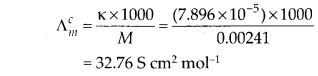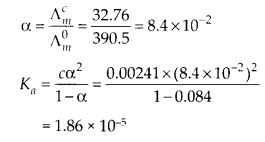Question 12.
How much charge is required for the following reductions:

1. 1 mol of Al3+to Al ?
2. 1 mol of Cu2+ to Cu ?
3. 1 mol of MnO4– to Mn2+ ?

Solution: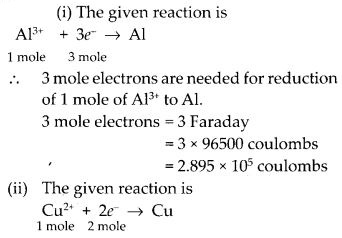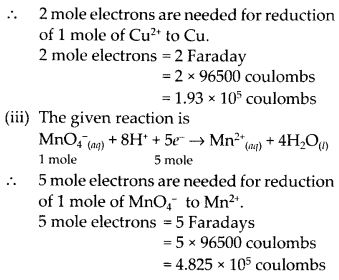Question 13.
How much electricity in terms of Faraday is required to produce

1. 20.0 g of Ca from molten CaCl2?
2. 40.0 g of Al from molten Al2O3?

Solution: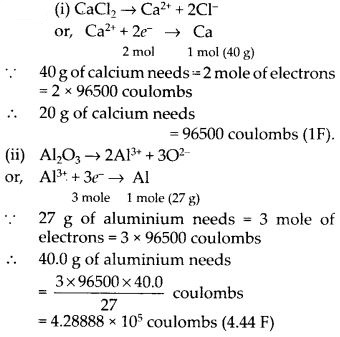Question 14.
How much electricity is required in coulomb for the oxidation of

1. 1 mol of H2O to O2?
2. 1 mol of FeO to Fe2O3?

Solution:Question 15.
A solution of Ni(NO3)2 is electrolysed between platinum electrodes using a current of 5 amperes for 20 minutes. What mass of Ni is deposited at the cathode?
(At. mass of Ni = 58.7)

Solution:Question 16.
Three electrolytic cells A, B, C containing solutions of ZnSO4, AgNO3 and CuSO4 respectively are connected in series. A steady current of 1.5 amperes was passed through them until 1.45 g of silver deposited at the cathode of cell B. How long did the current flow? What mass of copper and zinc were deposited?
(At. wt. of Ag = 108, Cu = 63.5, Zn = 65.3)

Solution: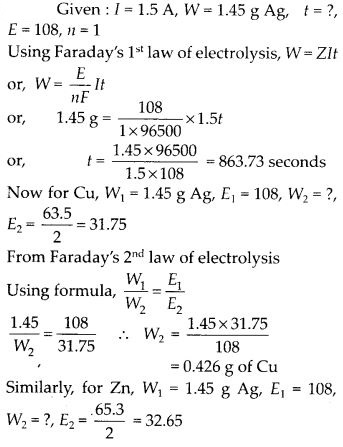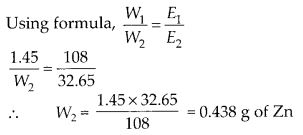Question 17.
Using the standard electrode potentials predict if the reaction between the following is feasible :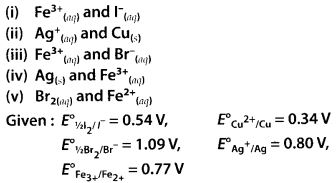Solution: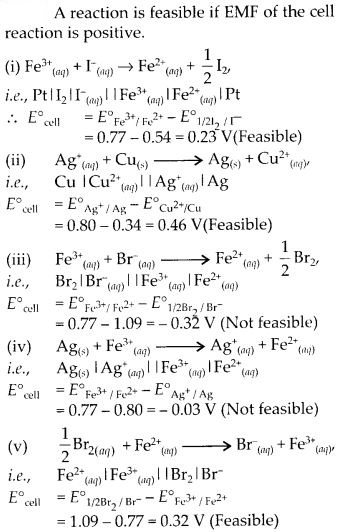Question 18.
Predict the product of electrolysis in each of the following:

1. An aqueous solution of AgNO3 with silver electrodes.
2. An aqueous solution of AgNO3 with platinum electrodes.
3. A dilute solution of H2SO4 with platinum electrodes.
4. An aqueous solution of CuCI2 with platinum electrodes.

Solution: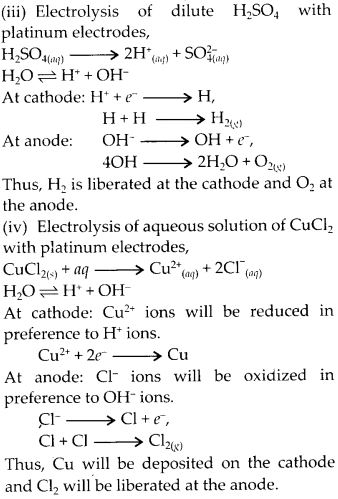All Chapter NCERT Solutions For Class12 Chemistry

—————————————————————————–

All Subject NCERT Solutions For Class12

*************************************************

Remark:

I think you got complete solutions for this chapter. If You have any queries regarding this chapter, please comment on the below section our subject teacher will answer you. We tried our best to give complete solutions so you got good marks in your exam.

If these solutions have helped you, you can also share Careerkundali.in to your friends.

Best of Luck!!